Next: Two recirculating filters for Up: Elementary filters Previous: Compound filters   Contents   Index

## Real outputs from complex filters

In most applications, we start with a real-valued signal to filter and we need a real-valued output, but in general, a compound filter with a transfer function as above will give a complex-valued output. However, we can construct filters with non-real-valued coefficients which nonetheless give real-valued outputs, so that the analysis that we carry out using complex numbers can be used to predict, explain, and control real-valued output signals. We do this by pairing each elementary filter (with coefficient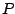or) with another having as its coefficient the complex conjugate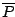or.

For example, putting two non-recirculating filters, with coefficientsand, in series gives a transfer function equal to: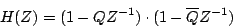which has the property that:Now if we put any real-valued sinusoid: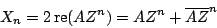we get out:which, by inspection, is another real sinusoid. Here we're using two properties of complex conjugates. First, you can add and multiply them at will: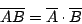and second, anything plus its complex conjugate is real, and is in fact twice its real part:This result for two conjugate filters extends to any compound filter; in general, we always get a real-valued output from a real-valued input if we arrange that each coefficientandin the compound filter is either real-valued, or else appears in a pair with its complex conjugate.Next: Two recirculating filters for Up: Elementary filters Previous: Compound filters   Contents   Index
Miller Puckette 2006-12-30